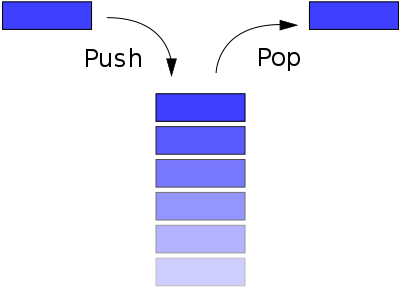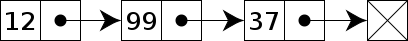# A Stack in Ruby using Linked Lists

published Jul 3, 2015

## What is a Stack?A Stack is an abstract data type in programming which has a variety of uses. The basic premise of a Stack is that you can add a new value to the end (pushing), and you can remove the last value off of the end. This is referred to as LIFO - Last In First Out.

Our Stack will have 3 external methods: `push` (aliased as `<<`), `pop` and `is_empty?`.

### An overview of our classes

Here is a quick high level overview of how the code will be structured.

`module LinkedList  class Node    # Linked List implementation  end  class Stack    # Stack methods reside here  endend`

## What is a Linked List?To build a Stack we need another data type to help us out. For this we can use an Array or a Linked List, which we'll be using in this article. A Linked List is a simple object (we'll call it a Node) which has its own value or data, plus a pointer to the next Node in the list. The last Node in the list points to `nil`.

`class Node  attr_accessor :value, :next_node  def initialize(value, next_node)    @value = value    @next_node = next_node  endend`

## Initializing the Stack

There isn't much to do to initialize things. Basically what we need to do is set a @first variable equal to nil.

`def initialize  @first = nilend`

## Pushing a new value to the Stack

To push a value to the Stack we'll want to create a new Node which has a next Node equal to the current first Node. Then we'll set the first Node equal to the new one we just created. Because Ruby is evaluated from right to left, we can do it in a single line of code.

`def push(value)  @first = Node.new(value, @first)end`

## Popping a value off the Stack

To pop a value off of the Stack we first want to check if we are already dealing with an empty Stack. If so we'll raise an exception.

Next we'll grab the value of the first Node, make the first Node equal to the next Node of the current first Node, and finally we'll return the value.

`def pop  raise "Stack is empty" if is_empty?  value = @first.value  @first = @first.next_node  valueend`

## Is the Stack empty?

This is the easiest question to ask. All we have to do is check whether the first Node is equal to nil. If so, the Stack is empty.

`def is_empty?  @first.nil?end`

## Final Version

Here is the final version of our Linked List Stack.

`module LinkedList  class Node    attr_accessor :value, :next_node    def initialize(value, next_node)      @value = value      @next_node = next_node    end  end  class Stack    def initialize      @first = nil    end    def push(value)      @first = Node.new(value, @first)    end    alias_method :"<<", :push    def pop      raise "Stack is empty" if is_empty?      value = @first.value      @first = @first.next_node      value    end    def is_empty?      @first.nil?    end  endend`

Here is how to use our Stack:

`stack = LinkedList::Stack.newstack << "The"stack << "Quick"stack << "Brown"stack << "Fox"begin  puts stack.pop  puts stack.pop  puts stack.pop  puts stack.pop  puts stack.poprescue RuntimeError => e  puts "Error - #{e.message}"end`

And here is the output it produces:

`# Fox# Brown# Quick# The# Error - Stack is empty`